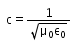JEE  >  Test: Characteristics of EM Waves

# Test: Characteristics of EM Waves

Test Description

## 10 Questions MCQ Test Physics Class 12 | Test: Characteristics of EM Waves

Test: Characteristics of EM Waves for JEE 2023 is part of Physics Class 12 preparation. The Test: Characteristics of EM Waves questions and answers have been prepared according to the JEE exam syllabus.The Test: Characteristics of EM Waves MCQs are made for JEE 2023 Exam. Find important definitions, questions, notes, meanings, examples, exercises, MCQs and online tests for Test: Characteristics of EM Waves below.
Solutions of Test: Characteristics of EM Waves questions in English are available as part of our Physics Class 12 for JEE & Test: Characteristics of EM Waves solutions in Hindi for Physics Class 12 course. Download more important topics, notes, lectures and mock test series for JEE Exam by signing up for free. Attempt Test: Characteristics of EM Waves | 10 questions in 10 minutes | Mock test for JEE preparation | Free important questions MCQ to study Physics Class 12 for JEE Exam | Download free PDF with solutions
 1 Crore+ students have signed up on EduRev. Have you?
Test: Characteristics of EM Waves - Question 1

### The speed of electromagnetic waves in free space is given by. Here μ0 is ___________and ε0 is ________________ of free space.

Detailed Solution for Test: Characteristics of EM Waves - Question 1

μ0 is called the permeability of free space and similarly, ε0 is called the permittivity of free space.

Test: Characteristics of EM Waves - Question 2

### Which region in the electromagnetic spectrum will have the highest speed?

Detailed Solution for Test: Characteristics of EM Waves - Question 2

All regions have the same speed.
The speed of a wave is not dependent upon its frequency and wavelength but rather upon the properties of the medium through which it travels.

Test: Characteristics of EM Waves - Question 3

### Which region in the electromagnetic spectrum has the highest frequency?

Detailed Solution for Test: Characteristics of EM Waves - Question 3

A gamma ray or gamma radiation is a penetrating electromagnetic radiation arising from the radioactive decay of atomic nuclei. It consists of the shortest wavelength electromagnetic waves and so imparts the highest photon energy

Test: Characteristics of EM Waves - Question 4

Electromagnetic waves constitute of

Detailed Solution for Test: Characteristics of EM Waves - Question 4

Maxwell predicted that an accelerated charge produces a sinusoidal time varying magnetic field, which in turn produces a sinusoidal time varying electric field. These two fields are mutually perpendicular to each other and sources of each other. They (fields) form an electromagnetic wave i.e. the wave that is propagated by simultaneous periodic variations of electric field and magnetic field intensity.

Test: Characteristics of EM Waves - Question 5

In electromagnetic waves the ratio of amplitudes of electric field and magnetic field is equal to​

Detailed Solution for Test: Characteristics of EM Waves - Question 5

In electromagnetic waves the ratio of amplitudes of electric field and magnetic field is equal to the velocity of the electromagnetic waves in free space.

(E/ B0) = c

Test: Characteristics of EM Waves - Question 6

Which statement is true about electromagnetic waves?

Detailed Solution for Test: Characteristics of EM Waves - Question 6

Properties:
• Electromagnetic waves are propagated by oscillating electric and magnetic fields oscillating at right angles to each other. Hence (a) is incorrect.
• Electromagnetic waves travel with a constant velocity of  3 x 108 ms-1 in vacuum. Hence (c) is incorrect.
• Electromagnetic waves need no medium of propagation. The energy from the sun is received by the earth through electromagnetic waves. Hence, correct answer is (b).

Test: Characteristics of EM Waves - Question 7

Which of the following is not a use of electromagnetic wave?

Detailed Solution for Test: Characteristics of EM Waves - Question 7

Electromagnetic waves are not used to determine global warming.

Test: Characteristics of EM Waves - Question 8

Which colour of the visible spectrum has the greatest wavelength?

Detailed Solution for Test: Characteristics of EM Waves - Question 8

Red light has the lowest energy and is least scattered and has thus least frequency, thus we get that it has the largest wavelength. Similarly violet has maximum frequency.

Test: Characteristics of EM Waves - Question 9

The electric field for an em wave is: E = E0sin[(12 x 1015 t – 4 x 107 x)]. What is the speed of the em waves?​

Detailed Solution for Test: Characteristics of EM Waves - Question 9

V(velocity/speed)= ω/wave number (K)
V= 12×1015/4×107
V=3×108 m/s

Test: Characteristics of EM Waves - Question 10

The wavelength of X-rays is of the order of:

Detailed Solution for Test: Characteristics of EM Waves - Question 10

X-ray wavelength is in range of 10−11m to 10−8m

So option C is correct.

## Physics Class 12

157 videos|452 docs|213 tests
 Use Code STAYHOME200 and get INR 200 additional OFF Use Coupon Code
Information about Test: Characteristics of EM Waves Page
In this test you can find the Exam questions for Test: Characteristics of EM Waves solved & explained in the simplest way possible. Besides giving Questions and answers for Test: Characteristics of EM Waves, EduRev gives you an ample number of Online tests for practice

## Physics Class 12

157 videos|452 docs|213 tests